# Search algorithm

(diff) ← Older revision | Latest revision (diff) | Newer revision → (diff)

on a graph, graph search

An algorithm that tries to find all nodes in a network or graph that satisfy a given property. Such algorithms are also used, e.g., to optimize a function on a graph or network or on a set which can easily be turned into a graph, such as. (Two-vectors of lengthare joined if an only if they differ in precisely one coordinate, yielding a hypercube.) Thus, these are definite relations and similarities between graph search and search theory as a systematic optimization technique (of enumeration type) [a3]. See [a4] for a very complete overview of global optimization (from the search point of view, including random search).

Two of the most often used search techniques in graphs or networks are depth-first search and breadth-first search. Letbe a finite oriented graph (cf. Graph, oriented). For each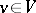, let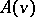be the set of edges (arcs) issuing from. For instance, in Fig.a1,,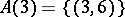,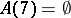.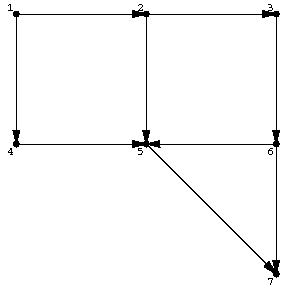Figure: s110070a

It is assumed that the sets,, are given some fixed total order (cf. also Totally ordered set) for all. The following algorithm picks out all nodes inthat are reachable from a source nodeby means of a directed path. These nodes will be marked. Central to the algorithm is a certain list, which can be seen as a sort of wave front indicating the boundary of the spreading blob of marked points:

unmark all points;

mark the source node;

set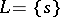;

as long asis non-empty, run the following iterative procedure:

choose a node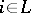;

select the first oriented edge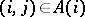that runs (from) to an unmarked node(if any);

mark the node;

define the functiononas;

addto;

if there is nothat runs to an unmarked node, removefrom.

stop whenis empty.

The algorithm does not yet specify how to select a node from. Ifis treated as a queue, i.e., the node selected is the one that has been longest in(first-in-first-out) the result is breadth-first search. On the other hand, ifis treated as a stack, i.e., the node selected is the one that was last added to(last-in-first-out) the result is depth-first search.Figure: s110070b

For an oriented tree as in Fig.a2 (downwards oriented), starting at the indicated node, breadth-first search will first pick the three children of the node indicated (in some order) and then will proceed to the next layer. Depth-first search will first pick a maximal downward chain (maximal in the sense that it ends at a leaf, not necessarily maximal in length) and then go back to another child of the last branching node in that chain.

The order which is used on the edge setsalso matters, but not as much.

The edges picked out by the function(reverse path), i.e., the edges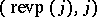form an oriented tree comprising the reachable nodes from the source node.

The results of the algorithm in four cases are given below for the case of Fig.a1.'

<tbody> </tbody>
 search marking oriented method sequence tree breadth first, 1 2 4 3 5 6 7lexicographic order on the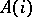breadth first, 1 4 2 5 3 7 6reverse lexicographic order on thedepth first 1 2 3 6 5 7 4lexicographic order on thedepth first 1 4 5 7 2 3 6reverse lexicographic order on theThere is an obvious analogous search algorithm for non-oriented graphs. These algorithms run intime.

 [a1] R.K. Ahuja, T.L. Magnanti, J.B. Orlin, "Network flows" G.L. Nemhauser (ed.) A.H.G. Rinnooy Kan (ed.) M.J. Todd (ed.) , Optimization , North-Holland (1991) pp. 211–370 [a2] H.M. Salkin, "Integer programming" , Addison-Wesley (1975) [a3] C.H. Papadimitriou, K. Steiglitz, "Combinatorial optimization" , Prentice-Hall (1982) [a4] A.A. Zhigljavsky, "Theory of global random search" , Kluwer Acad. Publ. (1991) (In Russian)
How to Cite This Entry:
Search algorithm. Encyclopedia of Mathematics. URL: http://encyclopediaofmath.org/index.php?title=Search_algorithm&oldid=16418
This article was adapted from an original article by M. Hazewinkel (originator), which appeared in Encyclopedia of Mathematics - ISBN 1402006098. See original article